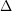## Discretization and simulation for a class of SPDEs with applications to Zakai and McKean-Vlasov equations

 Title Discretization and simulation for a class of SPDEs with applications to Zakai and McKean-Vlasov equations Publication Type Journal Article Year of Publication 2005 Authors Emmanuel Gobet, Gilles Pagès, Huyên Pham, and Jacques Printems Keywords Euler scheme, Malliavin calculus, Malliavin calculus., McKean-Vlasov equations, Nonlinear filtering, quantization, Stochastic partial differential equations, {E}uler scheme, {M}alliavin calculus., {M}c{K}ean-{V}lasov equations, {N}onlinear filtering, {S}tochastic partial differential equations Abstract This paper is concerned with numerical approximations for a class of nonlinear stochastic partial differential equations: Zakai equation of nonlinear filtering problem and McKean-Vlasov type equations. The approximation scheme is based on the representation of the solutions as weighted conditional distributions. We first accurately analyse the error caused by an Euler type scheme of time discretization. Sharp error bounds are calculated: we show that the rate of convergence is in general of order(is the time step), but in the case when there is no correlation between the signal and the observation for the Zakai equation, the order of convergence becomes. This result is obtained by carefully employing techniques of Malliavin calculus. In a second step, we propose a simulation of the time discretization Euler scheme by a quantization approach. This formally consists in an approximation of the weighted conditional distribution by a conditional discrete distribution on finite supports. We provide error bounds and rate of convergence in terms of the numberof the grids of this support. These errors are minimal at some optimal grids which are computed by a recursive method based on Monte Carlo simulations. Finally, we illustrate our results with some numerical experiments arising from correlated Kalman-Bucy filter and Burgers equation.